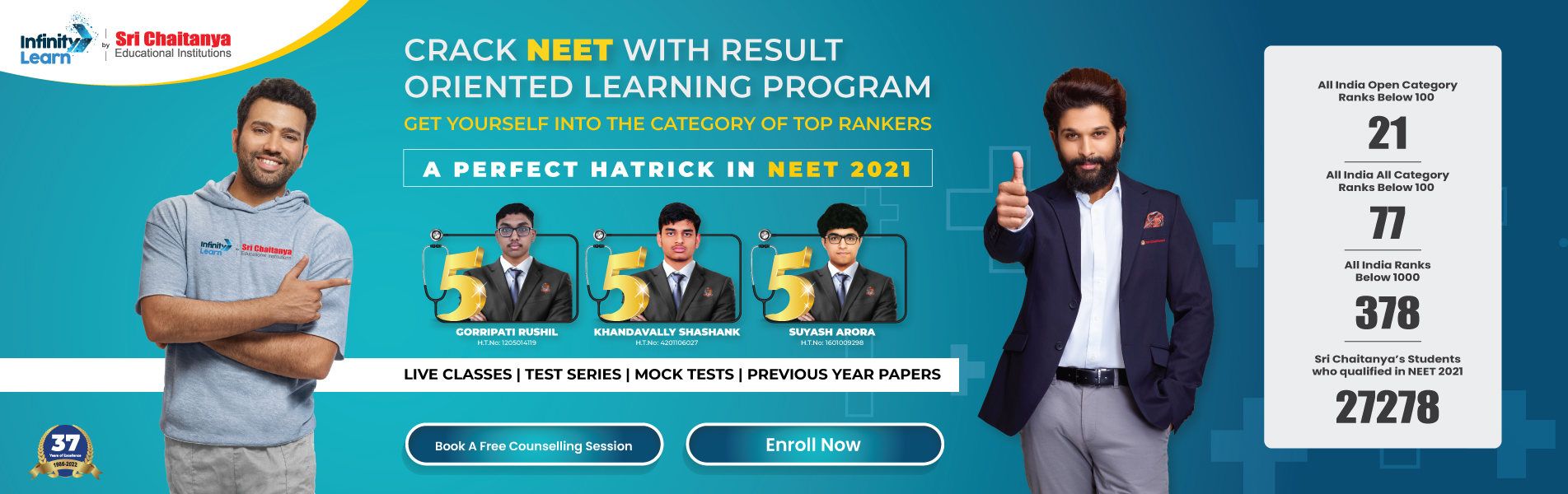NCERT Solutions for Class 12 Maths Chapter 6 Application of Derivatives

# NCERT Solutions for Class 12 Maths Chapter 6 Application of DerivativesInfinity Learn NEET IIT-JEE
Infinity Learn NEET
banner-chaitanya-web

Subject specialists have created NCERT Solutions for Class 12 Maths Chapter 6 Application of Derivatives, which includes thorough solutions for reference. All of the questions from the textbook’s exercises are answered here. Students can use these answers to help them prepare for their exams. The NCERT Solutions for Class 12 provide useful solutions for improving conceptual knowledge.

The solutions are carefully solved using student-friendly terms while still adhering to the norms that must be followed when solving NCERT Solutions for Class 12. Practicing these answers can be incredibly advantageous not only in exams but also in helping Class 12 pupils perform well in upcoming competitive exams.

Free IIT-JEE/NEET/NCERT/CBSE Material

Download 100,000+ FREE PDFs, solved questions, Previous Year Papers, Quizzes, and Puzzles directly to your Whatsapp account!

The approaches for answering have been given special consideration to stay on target while not deviating from the intended answer. Because time is so important in exams, excellent time management is essential for getting the best results when answering questions.

Do you need help with your Homework? Are you preparing for Exams? Study without Internet (Offline)
×
Chapter 6

## NCERT Solutions for Class 12 Maths Chapter 6 Application of Derivatives

The rate of change of values, increasing and decreasing functions, tangents and normals, approximations, maxima and minima, and many other subjects are covered in this chapter of NCERT Solutions for Class 12 Maths. The following are some key concepts:

1. A function f is said to be
1. increasing on an interval (a, b) if x1 < x2 in (a, b) ⇒ f(x1) < f(x2) for all x1, x2 ∈ (a, b)
2. decreasing on (a,b) if x1 < x 2 in (a, b) ⇒ f(x1 ) > f(x2 ) for all x1 , x2 ∈ (a, b).
3. constant in (a, b), if f (x) = c for all x ∈ (a, b), where c is a constant.
1. First Derivative Test
2. Second Derivative Test

The NCERT Solutions for Class 12 Maths Chapter 6 can be a quick reference to help students understand crucial and complicated topics.

## NCERT Solutions For Class 12 Maths Chapter 6 Exercises:

 Maths Class 12 NCERT Solutions Chapter 6 Exercises Exercise 6.1 Class 12 Maths Exercise 6.2 Class 12 Maths Exercise 6.3 Class 12 Maths Exercise 6.4 Class 12 Maths Exercise 6.5 Class 12 Maths Miscellaneous Exercise on Chapter 6 Class 12

### Key Features of NCERT Solutions for Class 12 Maths Chapter 6 Application of Derivatives

1. NCERT Solutions will aid in the development of student confidence.
2. The content is well-structured to make learning and understanding easier for students.
3. The NCERT Solutions are based on the most recent CBSE curriculum update for 2021-22.
4. This chapter will assist students in strengthening their understanding of derivatives.
5. Students can use NCERT Solutions to help them with their assignments and competitive exams.

#### Frequently Asked Questions on NCERT Solutions for Class 12 Maths Chapter 6

Why should I download Infinity learn NCERT Solutions for Class 12 Maths Chapter 6?

Answer: There are various reasons why you should download Infinity Learn’s NCERT Solutions for Class 12 Maths Chapter 6 created by specialists.

1. The CBSE board advises students to use the NCERT textbooks because they are one of the greatest study materials for exams.
2. NCERT Solutions are important since they provide answers to all of the questions in the NCERT textbook in one spot.
3. Infinity Learn’s subject matter specialists and teachers deliver solutions to assist students in performing well on term-wise exams.
##### What are the main applications of derivatives in our daily life according to NCERT Solutions for Class 12 Maths Chapter 6?

Derivatives are used to calculate the rate of change, growing or decreasing intervals, Lagrange’s Mean Value Theorem, Rolle’s Theorem, the point at which a tangent is parallel or perpendicular, the slope of a tangent or normal, and the approximate value of numbers, among other things. Our professionals explain these applications in simple and straightforward terms to help students grasp the key principles. Several examples are presented beneath each application to help students develop their conceptual understanding, regardless of their cognitive quotient.

Join Infinity Learn Regular Class Programme!